# Highlight specific elements in your charts with gghighlight

The `gghighlight` package in R is an extension of the ggplot2 package, designed to simplify the process of highlighting elements.
This post showcases the key features of `gghighlight` and provides a set of graph examples using the package.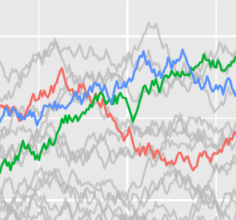{gghighlight}

# Quick start

The `gghighlight` package in R is an extension of the ggplot2 package, designed to simplify the process of highlighting elements.

It mainly offers a `gghighlight()` function that highlights geoms.

✍️ author → Hiroaki Yutani

📘 documentationgithub

⭐️ more than 500 stars on github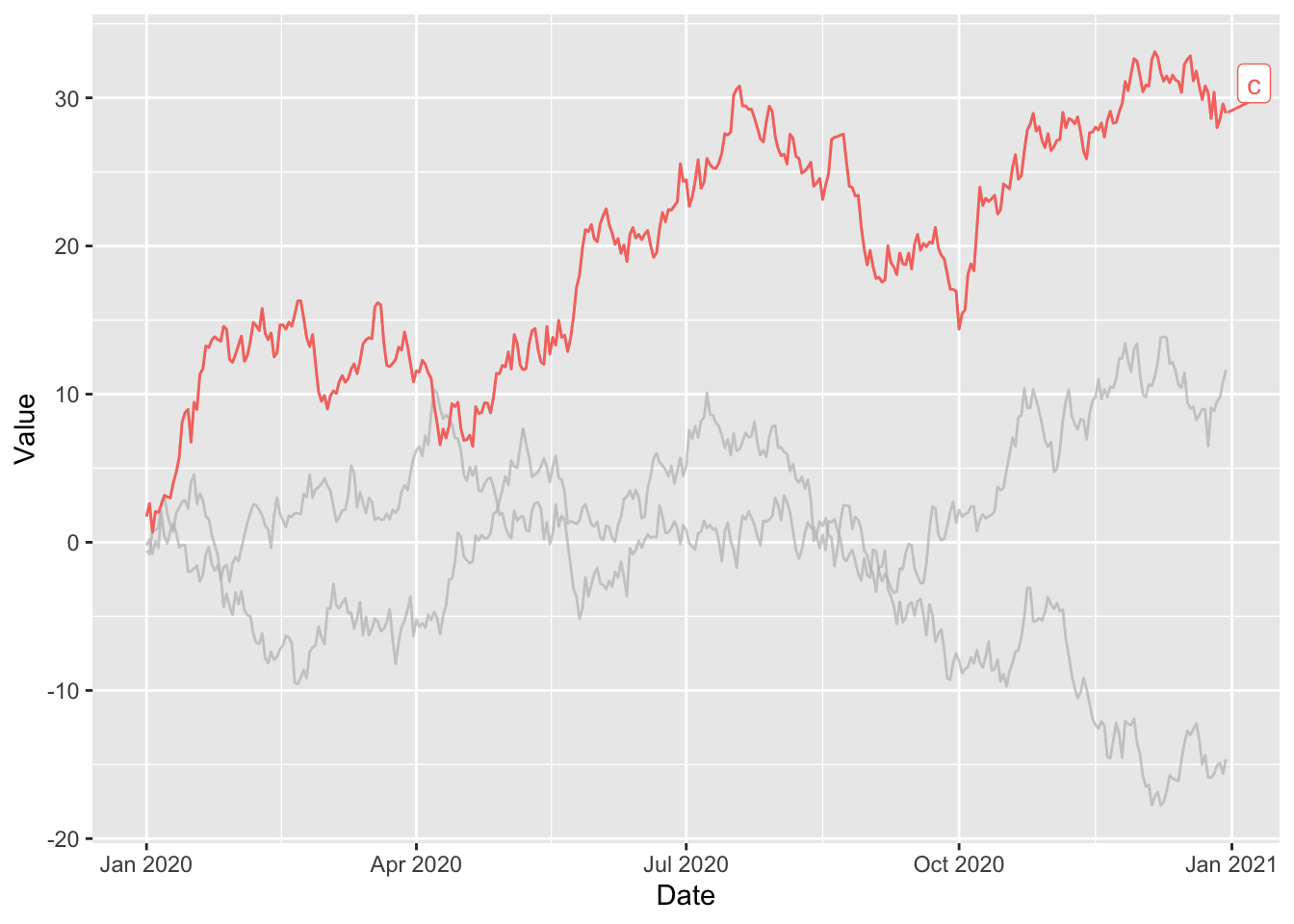# Installation

To get started with `gghighlight`, you can install it directly from CRAN using the `install.packages` function:

``install.packages("gghighlight")``

# Basic usage

The `gghighlight` package allows you to showcase specific elements in your ggplot2 charts.

Here’s a basic example on a line charts with multiple lines. At the end of the usual `ggplot2` call, the `gghighlight()` function is called with a single argument.

This argument is the condition that a group should respect to be highlighted.

``````library(ggplot2)
library(gghighlight)

# dataset with 3 lines named "a", "b" and "c"
set.seed(1)
period <- 365
df <- data.frame(
Date = seq(as.Date("2020-01-01"),
by = "day",
length.out = period
),
Value = c(
cumsum(rnorm(period)),
cumsum(rnorm(period)),
cumsum(rnorm(period))
),
Type = c(
rep("a", period),
rep("b", period),
rep("c", period)
)
)

ggplot(df) +
geom_line(aes(Date, Value, colour = Type)) +
gghighlight(min(Value) < -15) # highlight the group if its min value is below -15``````# Key features

## → Highlight a histogram with multiple groups

Use a facetted version with `facet_wrap(~ Species)` and the gghighlight feature allow us to showcase distribution of each group while maintaining the background distributions for other variables.

Example:

``````library(ggplot2)
library(gghighlight)

data(iris)

# show distribution of Sepal.Length of each species
ggplot(iris, aes(Sepal.Length, fill = Species)) +
geom_histogram() +
gghighlight() +
facet_wrap(~Species)``````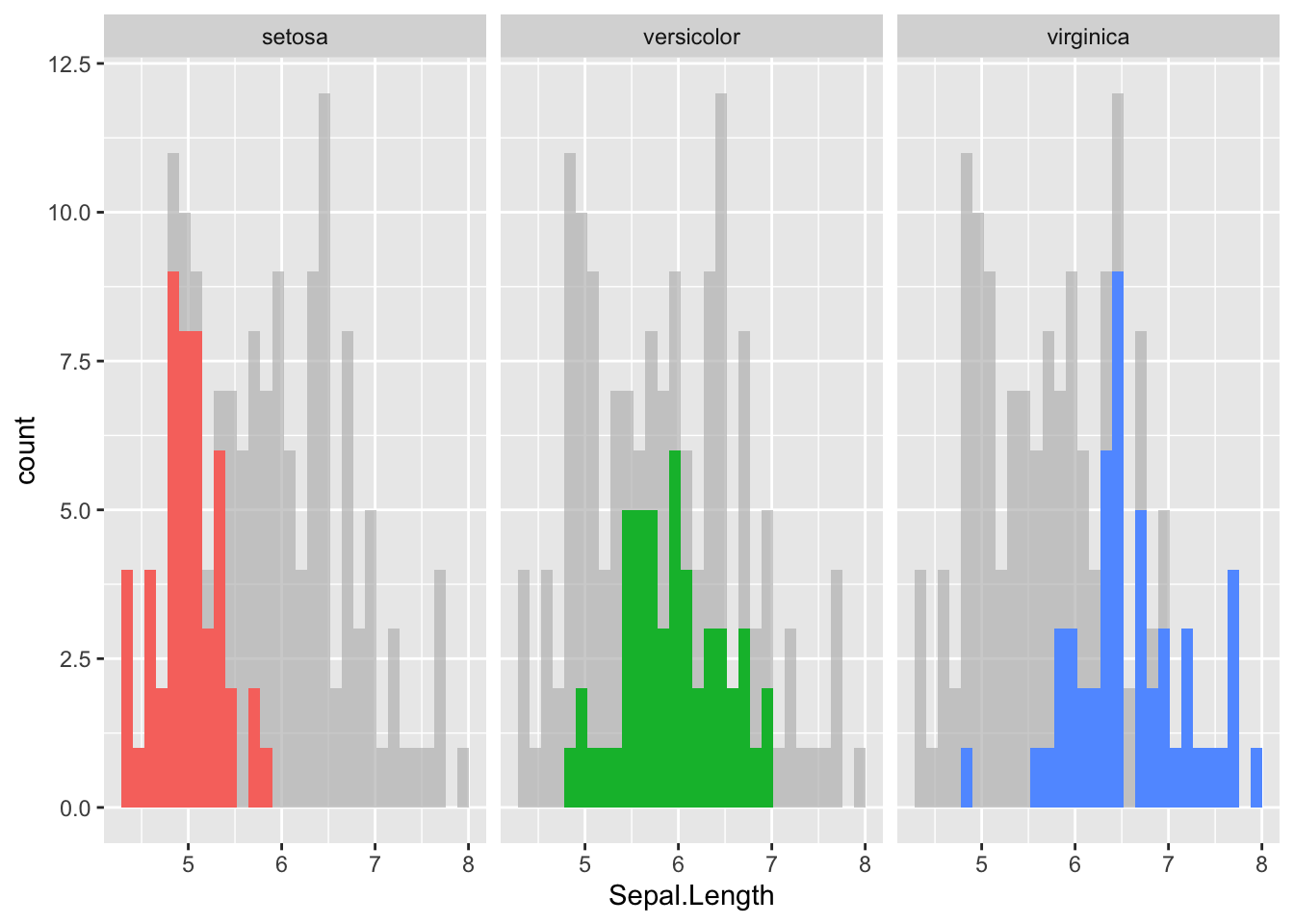## → Highlight points in a scatter plot

You can highlight specific points by indicating the properties of the points in question.

Example:

``````library(ggplot2)
library(gghighlight)

# create dataset
set.seed(1)
df_scatter <- data.frame(
xvalue = rnorm(n = 100),
yvalue = rnorm(n = 100)
)

# show distribution of Sepal.Length of each species
ggplot(df_scatter, aes(xvalue, yvalue)) +
geom_point() +
gghighlight(yvalue > 1.5, xvalue < 1)``````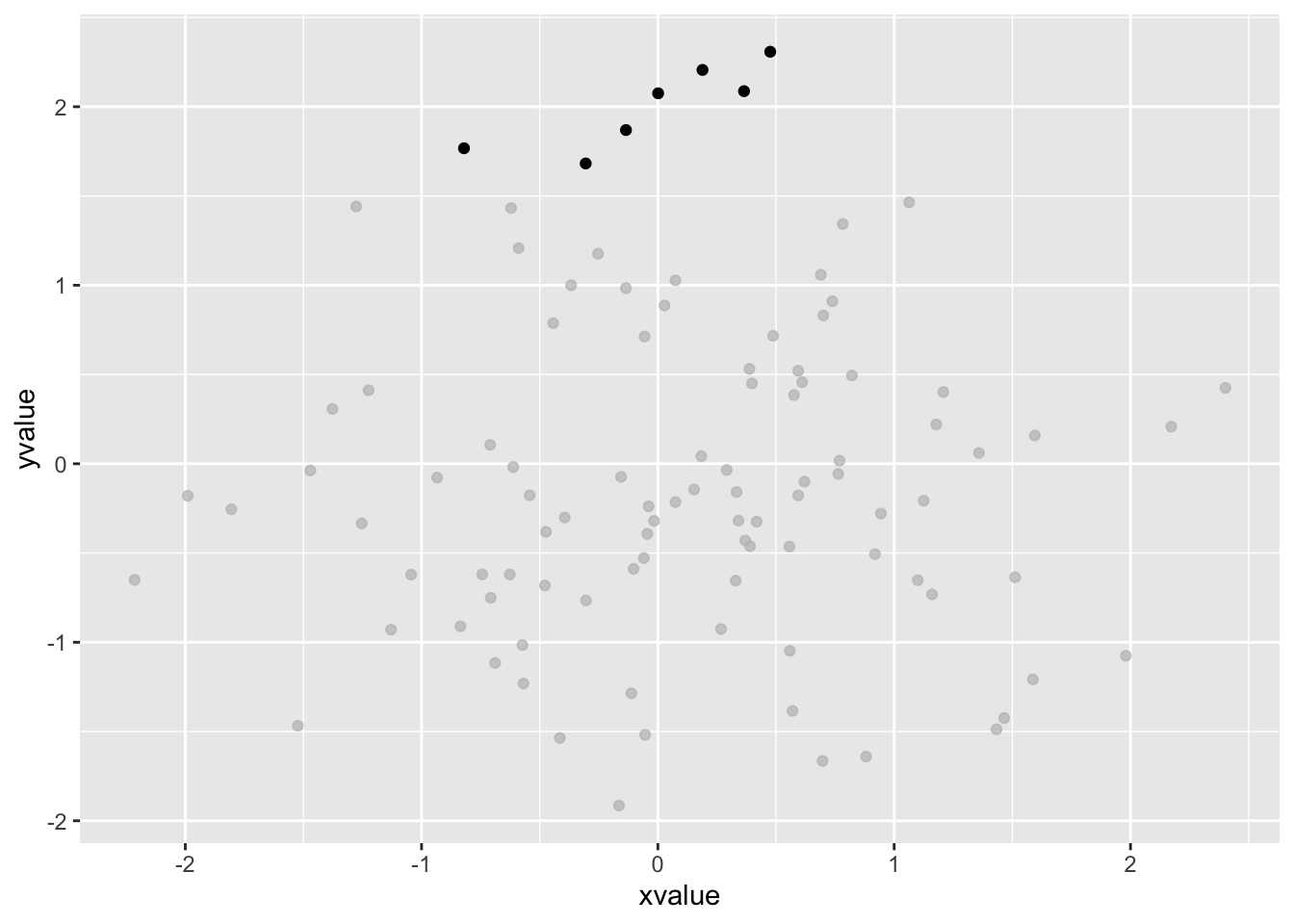## → Specify properties of unhighlighted

You can define the style of elements outside your filters using the `unhighlighted_params` argument.

Example:

``````library(ggplot2)
library(gghighlight)

ggplot(df) +
geom_line(aes(Date, Value, colour = Type)) +
gghighlight(min(Value) < -15,
unhighlighted_params = list(
linewidth = 0.3,
colour = alpha("lightblue", 0.8)
)
)``````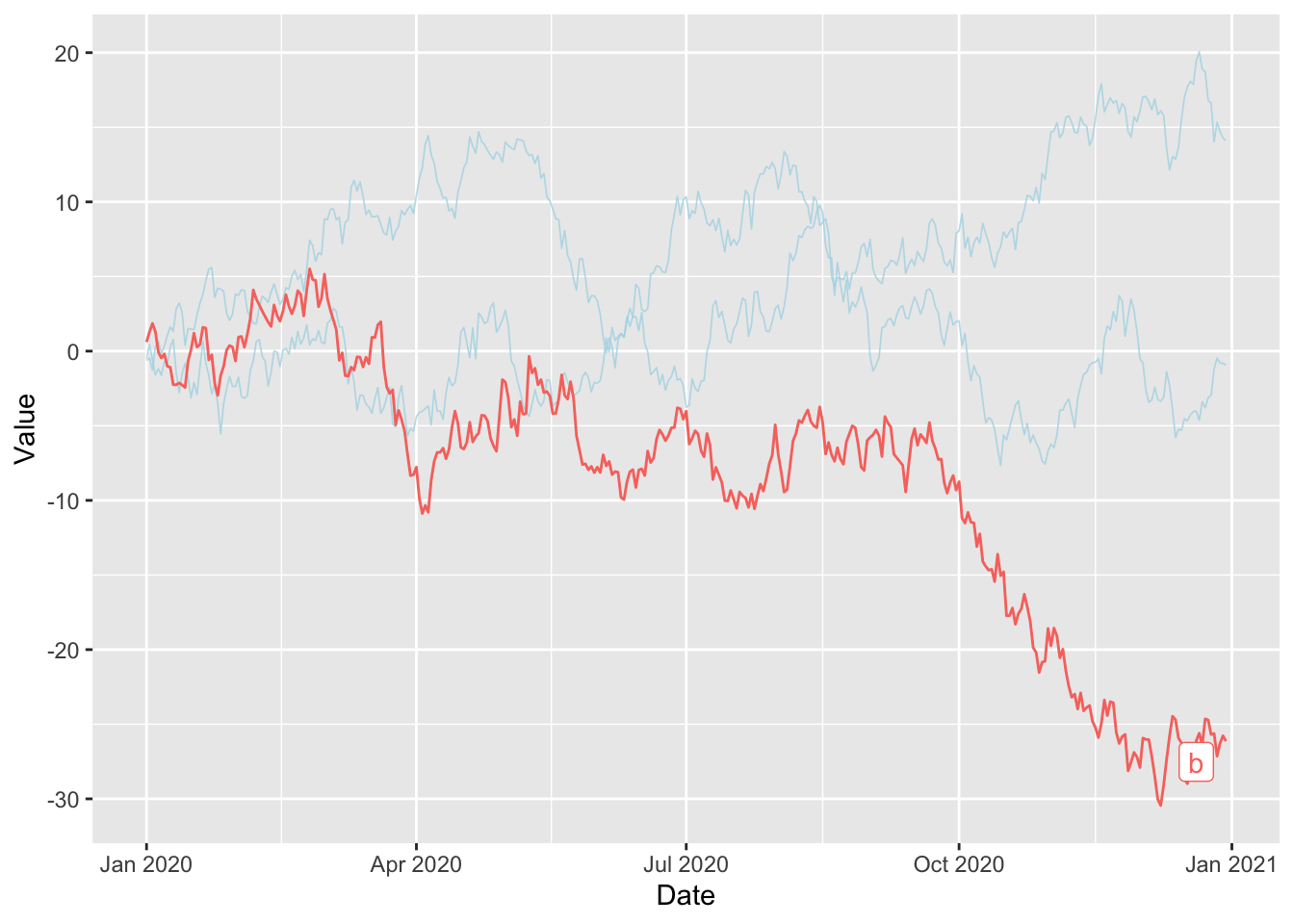## Contact

This document is a work by Yan Holtz. Any feedback is highly encouraged. You can fill an issue on Github, drop me a message on Twitter, or send an email pasting yan.holtz.data with gmail.com.